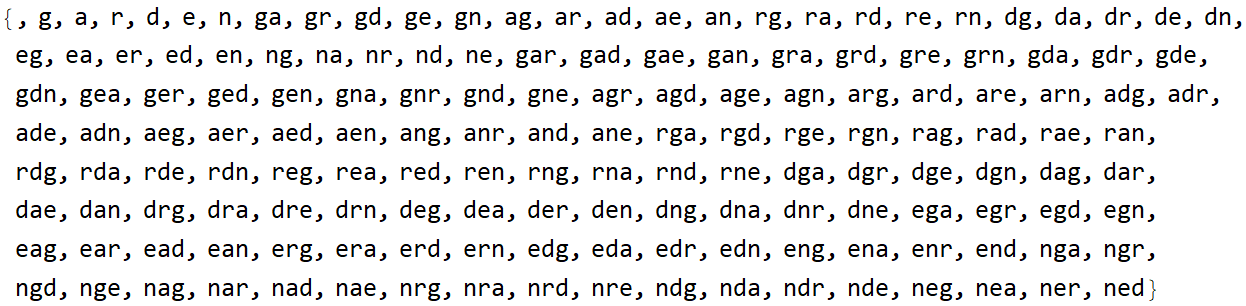# Wolfram CloudResource Object

Function Resource

# StringPermutations

Gives the specified character permutations for a string

 ResourceFunction["StringPermutations"][string] generates a list of all possible permutations of the characters in string. ResourceFunction["StringPermutations"][string,n] gives all permutations containing at most n characters. ResourceFunction["StringPermutations"][string,n] gives all permutations containing exactly n characters.

## Details and Options

StringPermutations is implemented with Characters and Permutations.
StringPermutations is the string analog of Permutations of a list.

## Examples

### Basic Examples

Permutations of "cat":

 In:=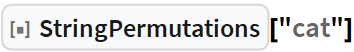Out=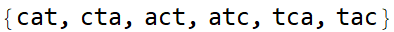Three character permutations of "horse":

 In:=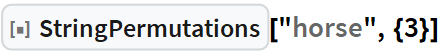Out=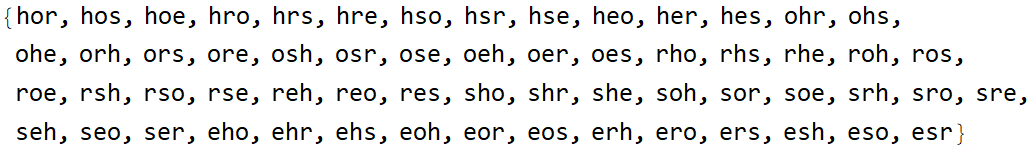One, two, and three character permutations of "garden":

 In:=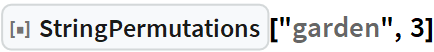Out=